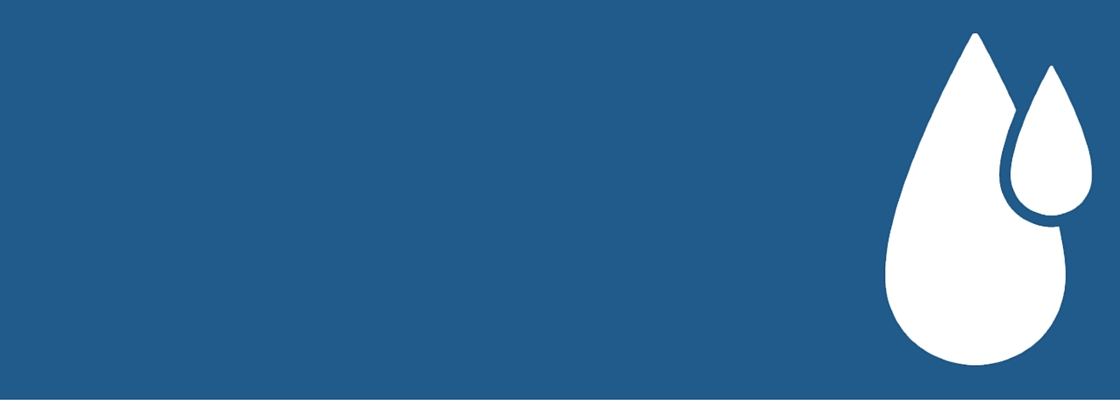# How To Calculate A Parts Per Million (ppm) Solution Of Sodium Hypochlorite (Hypo, Chlorine) Into A Simple Dilution Rate

Here’s a great question asked by one of our clients recently…

“Hi guys, I have received the order of hypochlorite solution, but I have a question with regards to dilution. The instructions I have are to mix this to a 200 parts per million solution. Can you please advise how much hypochlorite solution this would be? And to how much water? Thank you, Crystal”

For those of you who would just like to know how to quickly calculate cleaning chemical dilution rates and ratios click for the article here. Now let's start talking parts per million and dilution rates.

Our Hypo product label states that for general cleaning you should use at 1 part Hypo to 500 parts water, so for every 500 litres of ready to use solution one litre of Hypo needs to be added. General cleaning is the application we supply our Hypo for primarily but in many specialty applications where sanitising is required a parts per million recommendation or requirement can be requested.

Parts per million with a Hypo solution can be a difficult thing to accurately calculate and measure because by nature the Sodium Hypochlorite level in the concentrated form reduces over time from the point of manufacture. When manufactured the concentration is about 13% but this reduces due to time, exposure etc. For accurate instructions you are best consulting with whoever is recommending any specific direction as to how best to calculate the correct dilution to avoid any confusion because these matters are often critical in certain applications.

To answer Crystal’s question, it's not as simple as 200 parts Hypo to 1,000,000 parts Water (which would be 1:5000) because you have to allow for the starting concentration of Sodium Hypochlorite of 10-13%. Most of the time calculations are made at the 12.5% mark.

You should get your own advice but this is how I work it out…

To prepare 100 litres of a 200ppm solution from a 12.5% sodium hypochlorite (NaOCI) solution, the following calculations are needed…

###### Here’s the 3 important numbers you need before you start…

1. Final chlorine solution volume = 100 litres = 100,000ml (because 1 litre = 1000ml)

2. Final chlorine solution concentration desired = 200ppm

3. Initial chlorine solution concentration = 12.5% solution = 125,000/1,000,000 which can also be expressed as 125,000 parts per million (ppm) because 1ppm = 1ml in 1,000,000ml

###### So how much Hypo chlorine solution by volume (ml) do you need to add = ?

• Initial Hypo chlorine solution concentration x Initial Hypo chlorine solution volume (?) = Final chlorine solution volume x Final chlorine solution concentration desired

• 125,000ppm x ? = 100,000ml x 200ppm (Sorry, but a little bit of basic algebra is required)

• ? = 160ml.

So to prepare 100 litres of a 200ppm solution of Hypo – Sodium Hypochlorite, dilute 160ml of a 12.5% Sodium Hypochlorite solution with water

Hope this helps, you might find some online calculators through Google to make this easier so you don’t have to work it out each time.